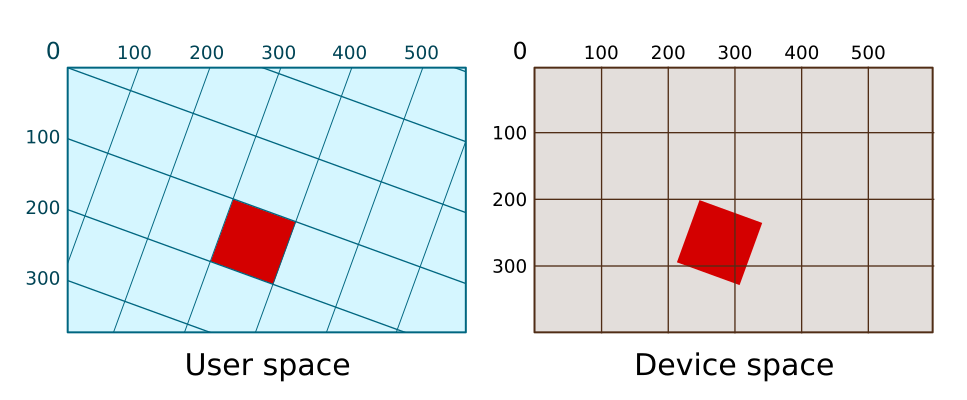# Transforms in Pycairo - rotate

By Martin McBride, 2019-09-27
Tags: transform rotation user space device space
Categories: pycairoWe have previously seen how to use translate and scale in Pycairo, to draw items in different positions on the page. In this article we will see how to use rotation to draw items at different angles.

## Default user space

Here is our initial square in default user space, with no transform applied.

ctx.rectangle(300, 100, 100, 100)
ctx.set_source_rgb(0, 0, 1)
ctx.fill()


This defines the rectangle in user space. Pycairo then draws the square in device space. Because of the default mapping, the square is drawn at exactly the same size and place in device space:Like many graphics libraries, Pycairo measures angles in radians rather than degrees. You can convert from degrees to radians by multiplying by pi/180.

So, for example, 20 degrees is equal to 20*pi/180 radians (ie about 0.35 radians).

## Rotating

The rotate function rotates user space. Here is an example:

ctx.rotate(20*math.pi/180)   # 20 degrees
ctx.rectangle(300, 100, 100, 100)
ctx.set_source_rgb(0, 0, 1)
ctx.fill()


This rotates user space by a 20 degrees. The whole of the user space is rotated. The centre of rotation is the point (0, 0).

Here is how the square is drawn using the rotated user space:The shape drawn is still a square, 100 pixel wide, but it is no longer aligned with the device space axes because it has been rotated.